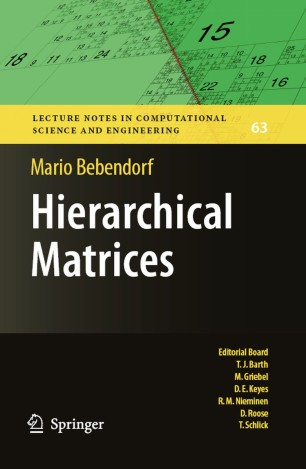# Hierarchical Matrices

## A Means to Efficiently Solve Elliptic Boundary Value Problems

• Mario BebendorfBook

Part of the Lecture Notes in Computational Science and Engineering book series (LNCSE, volume 63)

1. Front Matter
Pages i-xvi
2. Pages 1-7
3. Pages 49-98
4. Pages 99-192
5. Pages 193-267
6. Back Matter
Pages 269-296

### Introduction

Hierarchical matrices are an efficient framework for large-scale fully populated matrices arising, e.g., from the finite element discretization of solution operators of elliptic boundary value problems. In addition to storing such matrices, approximations of the usual matrix operations can be computed with logarithmic-linear complexity, which can be exploited to setup approximate preconditioners in an efficient and convenient way. Besides the algorithmic aspects of hierarchical matrices, the main aim of this book is to present their theoretical background. The book contains the existing approximation theory for elliptic problems including partial differential operators with nonsmooth coefficients.
Furthermore, it presents in full detail the adaptive cross approximation method for the efficient treatment of integral operators with non-local kernel functions.The theory is supported by many numerical experiments from real applications.

### Keywords

Approximation Boundary value problem approximation theory efficient algorithms in science and engineering numerical analysis partial differential equation partial differential equations scientific computing

#### Authors and affiliations

• Mario Bebendorf
• 1
1. 1.Mathematisches Institut Fakultät für Mathematik und InformatikUniversität LeipzigLeipzigGermany

Industry Sectors